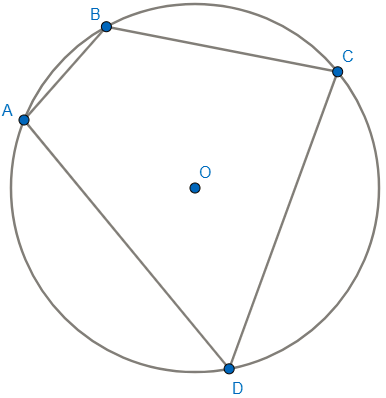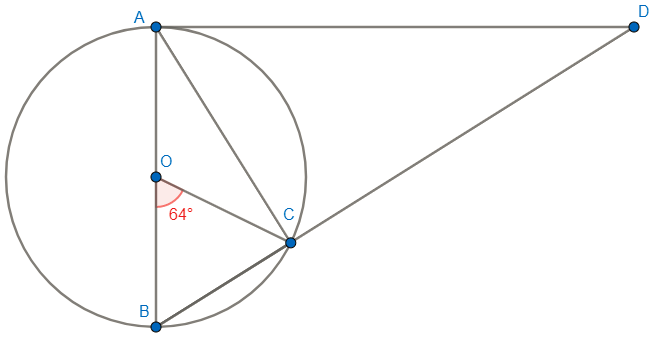Kindergarten
1st
2nd
3rd
4th
5th
6th
7th
8th
9th
10th
11th
12th
Higher Ed
Other
Subjects
ELA
Math
Science
Social Studies
Art
Computer Science
French
German
Music
Physical Education
Spanish
Other
Private Library
Practice Quiz 03.11 Inscribed Angles & Arcs
starstarstarstarstarstarstarstarstarstar
by Justin Nowell
| 7 Questions1
1 pt
Find the measure of angle C if the measure of angle A is 99 degrees.2
1 pt
Find the measure of angle D if the measure of angle B is 137.37 degrees.3
1 pt
Find the measure of angle A if angle A = 2x+18 and angle C = 3x+12.4
1 pt
Find the measure of angle D if angle B = 13r-12 and angle D = x+36.5
1 pt
Find the measure of angle A if angle A = 2x+26, angle B = 3x, and angle D = 57.6
1 pt
Find the measure of angle B if angle A = 17x, angle B = 13x, and angle C = 129.7
1 pt
SAT CHALLENGE PROBLEM: In this diagram, where O is the center of the circle, find the measure of angle D.

*** Remember this is a challenge problem like you would see on the SAT, NOT on your quiz tomorrow.***
A. 32
B. 36
C. 45
D. 58
E. 64
Add to my formatives list